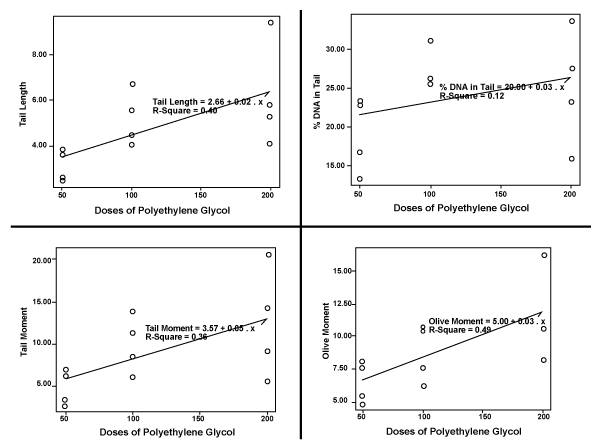Figure 2: The regression coefficients between polyethylene glycol concentrations and the comet assay parameters. (A) Tail length (r=0.40, p=0.03). (B) Tail DNA (r=0.12, p=0.27 (C) Tail moment (r=0.35, p=0.04). (D) Olive moment(r=0.49, p=0.01)